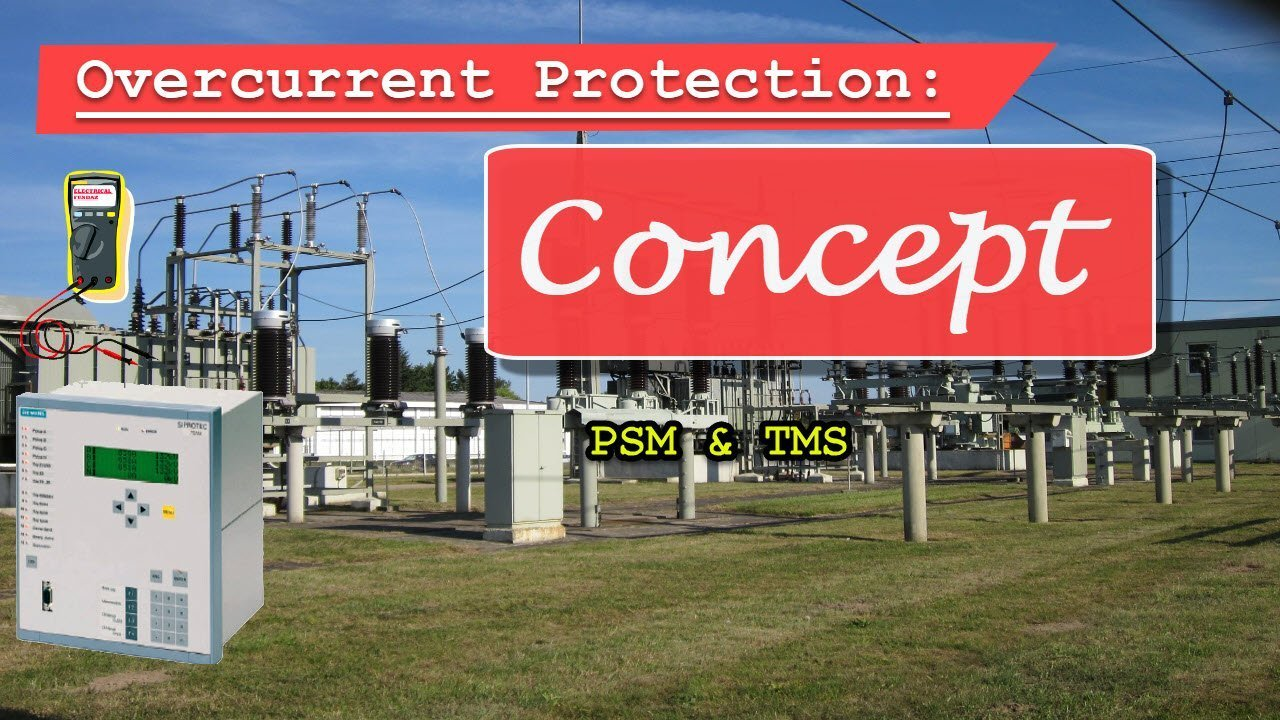In this post we will be seeing , the Basic Concept of Overcurrent Protection, which includes, relay Characteristics, Relay Settings with Example.

### What is an Overcurrent Relay?

1. Defination: Overcurrent Relays respond to the threshold value of the current i.e. once the current through the relay goes, beyond the set Value, it operates
2. ANSI Code: Its ANSI Code is 50.

EHV system is an Interconnected Network System of Circuit Element, having more than one Voltage Source. Hence the Fault current may flow  to Fault point from both end of any meshed line element. Hence,   simple Overcurrent Relays cannot provide proper Co-ordination and Discrimination for Meshed Transmission System.

Input to the Overcurrent Relay is Current (fed through CT) and Output is the Trip Command to the Master Trip Relay or the Breaker.
It has a Current Setting (PSM) which is the Actuating Quantity controlling the Operation of the Relay and Time Setting (TMS), which controls Operating Time of the Relay.
When the Relay Operates, it gives Trip Signal to the Master Trip Relay or directly to the Breaker.

What is Pick up of the Relay?
The value of the current at which the Relay Operates is called as Pickup Value of the Current. It is used in the terms of Plug Setting Multiplier.

In this type of Relays, Pick up current is the same and the time setting of a series of relays is such that the Breaker nearest to the fault opens first.
Major disadvantage of such type of grading is that, the Fault nearest to the Source (which has maximum current) is cleared after the longest delay.

In this type of Relays, Relay Operating time is the same and the Pick up of a series of Overcurrent relaysis chosen based on the assumed fault current which varies with the position of the Fault because of the difference of the Impedance values between the source and the Fault.
Major disadvantage of such type of grading is applicable only if there is large difference in magnitude of Fault current between the two Overcurrent relay locations, which is almost impossible to achieve.

### 1) Connected CT Ratio (CTR):

Lets say, CTR = 200/1

### 2) Maximum Load Current in Secondary (Imax sec)

It is the Maximum Current flowing through the Transmission Line , for the last Six Months or Full Load Current in case of Transformer .
Lets say, Imax = 150 A
So, Imax (sec) = 150/200 = 0.75 A

### 3) Relay Pickup Current, Ipu:

It is the current which when flows through the Relay Coil, the Relay Operates.
It is generally set at 120% of Maximum Load Current.

Ipu = 1.2 x Imax sec= 1.2 x 0.75 = 0.9 A

### 4) Relay Nominal Current (In):

Should be kept equal to CT Secondary Rated Current. Generally, it is 1A or 5A.
Lets say In = 1 A

### 5) Current Setting/Plug Setting (PS)

It is the Percentage of the Relay Nominal Current (In), which when flows through the Feeder, the Relay should Operates.
PS = Ipu sec / In = 0.9 / 1 = 0.9

### 6) Fault Current in Secondary (Ifsec):

For Overcurrent Setting we consider Three Phase Fault Current and for Earth Fault we consider , Single Phase Fault Current.
Say, If = 4000 A
If sec = If / CTR = 4000/200 = 20

### 7) Plug Setting Multiplier (PSM):

It is the Fault current seen by the relay in the Multiples of Plug Setting.

PSM= If sec / PS = 20/ 0.9 = 22.22

Note: PSM is used for time Calculation only and not required to be entered in the Relay Setting.

### 8) Time Margin (TM):

For 10C3 IDMT Curve, Time Margin is given by the Formula,

TM = 3 / Log PSM = 3 / Log 22.22 = 2.23

### 9) Relay Opersting Time (ROT):

It is the Time taken by the Relay to Operate and is given by the Formula,

ROT = TM x TMS

Where, TM is the Time Margin, that we have Calculated in Step 8.

### 10) Time Multiplier (TMS):

TMS is the Time Multiplier Setting which needs to be entered in the Relay Settings.

TMS = ROT/ TM

Lets say we want Relay to Operate in 450 ms I.e ROT = 450 ms

Then, TMS = 0.45 / 2.23 = 0.202, which needs to be entered in the Relay as the Time Setting.